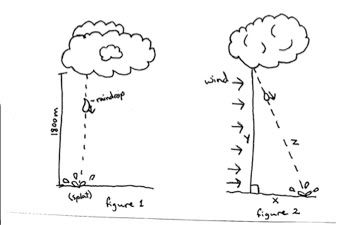×#### Thank you for registering.

One of our academic counsellors will contact you within 1 working day.

Click to Chat

1800-1023-196

+91-120-4616500

CART 0

• 0

MY CART (5)

Use Coupon: CART20 and get 20% off on all online Study Material

ITEM
DETAILS
MRP
DISCOUNT
FINAL PRICE
Total Price: Rs.

There are no items in this cart.
Continue Shopping
```
According to V=(2gh) 1/2 ,an object falling from a great height should have a very high velocity. That means a raindrop and a hailstone should hit the ground very hard with a terrible speed.But why doesnt that happen?

```
11 years ago147 Points
```							Hi Chilukuri Kartik
That relation V=(2gh)1/2 is drive for ideal case.
in derivation of this formula we have not consider the effect of drag forces.
Drag force acts in the reverse direction of the movieng particle.the more the velocity the more will be drag force.so it reduce the velocity of moving particle..
Thats why a raindrop and a hailstone should  not hit the ground very hard

Please feel free to post as many doubts on our discussion forum as you can. If you find any question Difficult to understand - post it here and we will get you the answer and detailed solution very quickly.  We are all IITians and here to help you in your IIT JEE preparation.    All the best Chilukuri Kartik       Regards,   Askiitians Experts   Badiuddin
```
11 years ago
```							Here lets refer to the kinetic energy of a raindrop that gains through transforming its gravitational potential energy as it falls from the clouds into force. rain usually form under 6000 feet, or about 1800 meters and average mass of a raindrop, 6.5×10^(-5) kg.
Next calculate the velocity the raindrop which would attain by falling from 1800 meters, first by calculating the time it would take to hit the ground, then finding the velocity as follows (see figure 1 for an illustration):  y = yinitial + vinitial t - .5gt^2  0 = 1800 m + 0t - .5(9.8 m/s^2)t^2  4.9t^2 = 1800  t^2 = 367.3  t = 19 s  v = vinitial + at    v = 0 - 9.8 m/s^2 (19 s)  v = -186m/s  or about -190 m/s  The raindrop was sure moving fast when it hit the ground, but how much force did it have?  KE = 0.5 mv^2  KE = 0.5 (6.5 × 10^(-5) kg) (-186 m/s)^2  KE = 1.1244 N or about 1.1 N  So, a raindrop does not have that much force, less than a fourth of a pound, and splats on impact anyway, but if it was a hailstone instead it would probably sting. When the wind is blowing, rain seems hit harder than when it is falling straight down. Is that just an impression from the weather being stormy, or reality? To find out, lets chose an average wind speed, 10 meters per second. To find out the velocity before impact of the raindrop, lets take the descent velocity and the wind speed, assuming the wind remains constant, for the y and x components, respectively, then used the Pythagorean Theorem to find z, or the velocity of a raindrop being driven by wind (see figure 2). we can then find the force of impact.  y = -186 m/s		x = 10 m/s  z = [ (-186 m/s)^2 + (10 m/s)^2]^(1/2)  z = 186.2686 m/s  KE = .5 ((6.5 × 10^(-5) kg) (186.2686 m/s)^2  KE = 1.1276 N or about 1.1 N  So, the force is not increased substantially. What if it was during a big storm with winds of 30 meters per second, about 66 miles per hour?  y = -186 m/s		x = 30 m/s  z = [ (-186 m/s)^2 + (30 m/s)^2]^(1/2)  z = 188 m/s  KE = .5 ((6.5 × 10^(-5) kg) (188 m/s)^2  KE = 1.15 N or about 1.2 N  Thus, there is about a 9% increase in force, which still is not much, but again, if it was hail instead, it would matter. This little foray into the impacts of raindrops makes me wish I could get my hands on a decent storm and some hail to see how it really would feel.--
regards
Ramesh
```
11 years ago
Think You Can Provide A Better Answer ?

## Other Related Questions on Mechanics

View all Questions »### Course Features

• 101 Video Lectures
• Revision Notes
• Previous Year Papers
• Mind Map
• Study Planner
• NCERT Solutions
• Discussion Forum
• Test paper with Video Solution### Course Features

• 110 Video Lectures
• Revision Notes
• Test paper with Video Solution
• Mind Map
• Study Planner
• NCERT Solutions
• Discussion Forum
• Previous Year Exam Questions